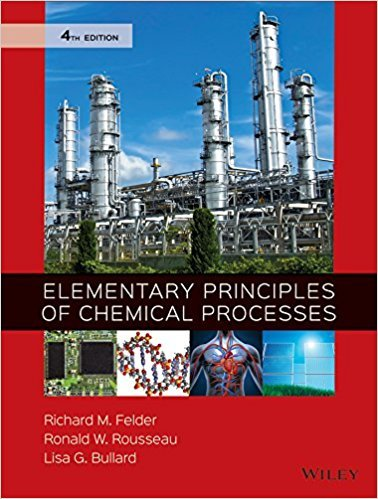×
×

# Solutions for Chapter 4.76: SUMMARY## Full solutions for Elementary Principles of Chemical Processes | 4th Edition

ISBN: 9780470616291Solutions for Chapter 4.76: SUMMARY

Solutions for Chapter 4.76
4 5 0 361 Reviews
23
3
##### ISBN: 9780470616291

Since 1 problems in chapter 4.76: SUMMARY have been answered, more than 41292 students have viewed full step-by-step solutions from this chapter. This expansive textbook survival guide covers the following chapters and their solutions. Chapter 4.76: SUMMARY includes 1 full step-by-step solutions. Elementary Principles of Chemical Processes was written by and is associated to the ISBN: 9780470616291. This textbook survival guide was created for the textbook: Elementary Principles of Chemical Processes, edition: 4.

Key Chemistry Terms and definitions covered in this textbook
• acetoacetic ester synthesis

A threestep process that converts an alkyl halide into a methyl ketone with the introduction of three new carbon atoms.

• alcohol.

An organic compound containing the hydroxyl group —OH. (24.4)

• chelating agent.

A substance that forms complex ions with metal ions in solution. (23.3)

• covalent bond

A bond that results when two atoms share a pair of electrons.

Reactions in which two p systems are joined together in a way that forms a ring. In the process, two p bonds are converted into two s bonds.

• dispersion forces

Intermolecular forces resulting from attractions between induced dipoles. Also called London dispersion forces. (Section 11.2)

• enthalpy

A measure of the exchange of energy between the system and its surroundings during any process.

• exergonic

Any process with a negative DG.

• gauche interaction

The steric interaction that results when two groups in a Newman projection are separated by a dihedral angle of 60°.

• greenhouse gases

Gases in an atmosphere that absorb and emit infrared radiation (radiant heat), “trapping” heat in the atmosphere. (Section 18.2)

• Hertz (Hz)

The unit in which frequency is measured: s 21 (read “per second”).

• homogeneous equilibrium

The equilibrium established between reactant and product substances that are all in the same phase. (Section 15.4)

• intermediate

A structure corresponding to a local minimum (valley) in an energy diagram.

• Keq

A term that describes the position of equilibrium for a reaction: Keq = 3H3O+ 4 3A- 4 3HA4 3H2O4

• momentum

The product of the mass, m, and velocity, v, of an object. (Section 6.4)

• photon

The smallest increment (a quantum) of radiant energy; a photon of light with frequency n has an energy equal to hn. (Section 6.2)

• pi (p) bond

A bond formed from adjacent, overlapping p orbitals.

• R

A term used to designate the configuration of a chirality center, determined in the following way: Each of the four groups is assigned a priority, and the molecule is then rotated (if necessary) so that the #4 group is directed behind the page (on a dash). A clockwise sequence for 1-2-3 is designated as R.

• Vinyl group

A carbocation in which the positive charge is on one of the carbons of a carbon-carbon double bond.

• zwitterion

A net neutral compound that exhibits charge separation. Amino acids exist as zwitterions at physiological pH.

×